## How Diffractive Surfaces are Modeled in OpticStudio

Category: Product NewsDiffractive optical elements (DOEs) are commonly used in moulded optics, specifically in IMPO and in chalcogenide PGM lenses for infrared imaging applications.

When working with diffractive elements in OpticStudio, it is important to understand how OpticStudio handles diffraction in ray tracing. This blog explains how diffractive surfaces are modeled, and the differences between kinoform and binary optics.

OpticStudio models diffractive power independent to the substrate index and the surface sag; diffractive power introduces phase change to rays. All diffractive surfaces in OpticStudio bends rays according to the following equation:Where:

• M is the diffraction order

• λ is the wavelength

• T is the grating period (inverse of the line spacing, d).

The equation above is Snell's law for refraction, plus an additional ray bending term representing diffraction. The diagram below shows the diffraction for a ray incident normally (sin(theta1) =0) for a diffractive surface with no refractive power.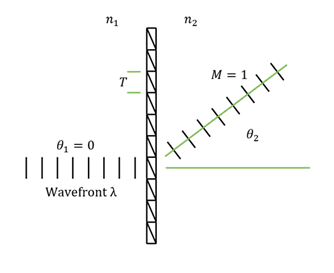A surface such as the Diffraction Grating surface has a constant period of grating lines along one axis and is commonly used in spectrometers. The real power of computer-generated diffractive surfaces is that the grating period can be made to vary spatially across the surface so that diffractive power can be added exactly where it is needed.

For example:

• The Variable Line-Spacing Grating surface allows the grating period to vary to model chirped gratings

• The Binary 1 surface allows the grating period to vary as an x-y polynomial expansion

• The Binary 2 surface allows the grating period to vary as a rotationally symmetric polynomial (this is very commonly used for aberration correction)

• A range of polynomial surfaces exist that use almost any polynomial expansion to describe the variation of grating spacing

• A Grid Phase surface allows an arbitrary set of {x,y} points to be used to define the phase added at any point

• A User-Defined surface can be written to describe any profile that is required, if a suitable surface type is not already available in OpticStudio

In all these cases, OpticStudio models the diffractive power as a phase profile on the surface. Rays are bent by the gradient of the phase profile introduced by the diffractive.

The phase φ in radians adds to the optical path length of the ray.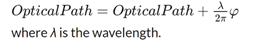The gradient of the phase profile (phase slope) change the direction of rays.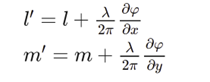where l is the x-direction cosine of the ray and m is the y-direction cosine of the ray.

The design process is therefore to compute the required phase profile, and then to compute the grating structure required to produce the phase profile.

A diffraction order has to be specified to each diffractive surfaces in the Lens Data Editor. Multiple diffraction orders can be modeled simultaneously via multiple configurations, as shown in the diagram below.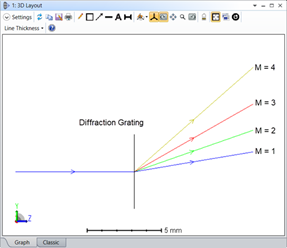According to the equation above, the diffraction angle depends only on the period (T) of the repetitive structure where the incident light hits, and not on the shape of the structure within that particular period. The surface structure does, however, affect the diffraction efficiency. OpticStudio can either model an ideal grating structure, where the efficiency at the specified diffraction order is assumed to be 100%, or use more sophisticated solvers to accurately compute the efficiency in each order from the surface structure. The latter approach is described in Zemax knowledgebase articles, Simulating diffraction efficiency of surface-relief grating using the RCWA method and Simulating diffraction efficiency of a volume holographic grating using Kogelnik’s method.

The sign of the diffractive order determines the sign of the diffraction angle with respect to the optical axis. The sign convention for the diffraction order is purely arbitrary. The convention used by OpticStudio is positive diffraction angles (with respect to the optical axis) for positive diffraction orders.

Diffractive surfaces in OpticStudio can have refractive as well as diffractive powers. The diffractive power introduces a continuous phase across the surface, according to their formula described in the manual. Since the phase is continuous, they represent ideal diffractive optical elements (DOE), where the period of the diffractive structure is infinitesimally small or at least very small compared to the wavelength.

Kinoform and binary diffractive surfaces

To maximize the diffraction efficiency in a DOE, the sag of the surface within the diffraction zones can be made such that the phase of the wavefront is parallel to the diffracted waves (of the desired diffraction order) everywhere. Figure 13.3 (b) shows a "blazed" transmission grating in which the blaze angle is optimized to maximize efficiency to a particular order .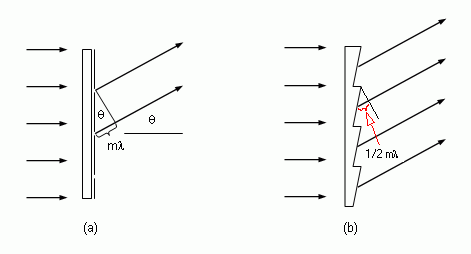A DOE with continuous surface profile shown in figure (b) above is often referred as kinoform. If the sag is approximated by discrete steps, as it is often the case when photolithography is used, it is commonly referred as a Binary Optic (see diagram below) . Ideal diffractive surfaces in OpticStudio are closer approximation to kinoforms than true binary optics, since the phase is continuous everywhere. It is up to the user to decide what surface structure to use to approximate the phase modeled by a diffractive surface.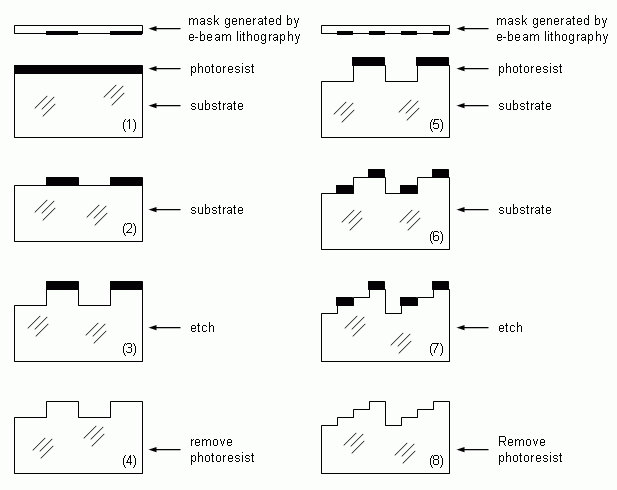The following diagram shows the theoretical efficiency of binary surface as a function of number of steps .